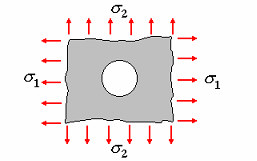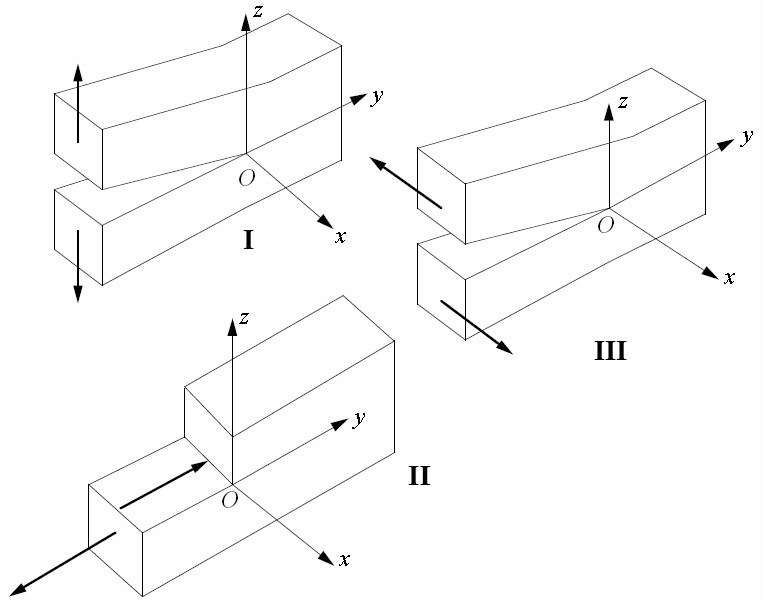# General Information

Quite a few structural elements feature certain geometrical singularities, for example, holes, notches, etc. Usually, maximum local stresses occur specifically on the boundaries of such singularities. This maximum local stress σmax is by far higher than the nominal stress σnom. The ratio of the maximum stress σmax to the nominal one σnom is known as the stress concentration factor Kt, that is,

σmax = Ktσnom

Suppose we deal with the flat stress problem. Depending on the way the nominal stress σnom is calculated — either by the total area of the given element (without distracting the singular object (hole) area) or that minus the singular object area — one distinguishes between the gross-area stress concentration factor (Ktg) and the net-area stress concentration factor (Ktn).

In some cases, for example, if we deal with holes in infinite plates (see Fig. 1), the concepts of gross-/ net-area stress concentration factors lose their significance, therefore one should refer only to the stress concentration factor Kt.Figure 1. Hole in an infinite plate

The studies of stress concentration are based on theoretical calculations, numerical analyses of various kinds, or experimental findings (photoelasticity). The results of numerous researches on stress concentration have been systematized and presented in the book by W.D. Pilkey Peterson’s Stress Concentration Factors. The implementation of CoCon is based largely on this publication.

CoCon deals with quite a few structural elements having various singularities and subjected to the action of one load (as a rule). In practice, most structural elements are subjected to the action of combined loads. In order to evaluate the maximum stress in these cases, it suffices to determine the maximum stresses for single loads using the nominal stresses and the single-load stress concentration factors, and then use the superposition principle. This is a possibility, since we assume the structure material to behave linearly and, in addition to that, the maximum stresses occur, as a rule, at the same points of a structure under different kinds of loading.

Moreover, CoCon offers the modes for calculating the stress intensity factors at the tip of a crack. Depending on a particular problem, the following three types of intensity factors can be calculated:

KI — intensity factors for the type I cracks (bond-failure cracks, or opening mode cracks);

KII — intensity factors for the type II cracks (transverse shear cracks);

KIII — intensity factors for the type III cracks (longitudinal, or out-of-plane shear cracks).Fig 2. Three types of cracks:

I — bond-failure cracks, II — transverse shear cracks, III — longitudinal shear cracks.